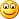## Integer compressionUsing SIMD bit packing in practice

time to read 3 min | 520 words

In the last post, I talked about how the simdcomp library is actually just doing bit-packing. Given a set of numbers, it will put the first N bits in a packed format. That is all. On its own, that isn’t very useful, we have to pair it with something else to make it do something more interesting.

Previously, I talked about delta compression, what if we put both of those together?

Given a sorted list of numbers, we’ll compute the delta between the numbers, then we can figure out how many bits we need to store them, and we can pack that tightly.

Analyzing the sample posting list I’m using, we get the following batches (of 128 numbers each)

• 328 batches at 13 bits
• 11 batches at 22 bits
• 7 batches at 23 bits
• 3 batches as 27 bits
• 1 batch at 31 bits
• 1 batch at 28 bits

Doing the math, we can fit all of those using 77Kb or so.

The idea is that for each batch, we can use simdpack() to write just the relevant bits. We’ll need some extra metadata to keep track of the number of bits per batch, but that is just one byte per every 128 values. For that matter, we can bit-pack that as well.

Note that in this case, we are using the simd packing routines just for bit packing. The actual compression aspect comes not from the packing, but from computing the deltas and then figuring out how densely we can pack those values.

This approach is called Frame Of Reference (FOR), and it has some really nice advantages. By splitting the data into blocks, we can take advantage of the patterns in the data. Here are the first 16 deltas in the posting list:

 Value Bits  1871143144 31  4 3  4 3  4 3  4 3  4 3  4 3  4 3  4 3  4 3  4 3  7984 13  4 3  4 3  4 3  4 3

Let’s assume that the batch size is 4 numbers, what would be the numbers here? Basically, we take the max number of bits for each batch of 4 numbers.

The first block will require us to use 31 bits, the second would be just 3 bits, but the third would require 13 bits and the last is 3 bits again.

The idea is that for each block, we have a separate frame of reference, the number of bits that are required for us to record & retrieve the data.

Of course, if you’ll look at the data itself, we are wasting quite a lot of space here needlessly. There are just two numbers (0, 11) that require more than 3 bits.

There is an algorithm called Patched Frame Of Reference (PFor) that handles this. Basically, when we compute a batch, we’ll record it using 3 bits only in this case. What about the rest of the values? Those we’ll store outside the batch, as an exception. On reading, we’ll need to patch the data to make it whole.

That adds a bit of complexity, but it also means that you can compress the data a lot more densely.

In the next post, I’m going to be talking about FastPFor, which combines simd bit packing, patched frame of references and some really clever tricks to get a very interesting integer compression algorithm.